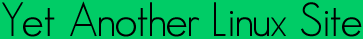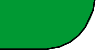Quick ?s
Cheat Sheets
Man Pages
The Lynx
Software
 ```FREXP(3) Linux Programmers Manual FREXP(3) NAME frexp, frexpf, frexpl - convert floating-point number to fractional and integral components SYNOPSIS #include double frexp(double x, int *exp); float frexpf(float x, int *exp); long double frexpl(long double x, int *exp); Link with -lm. DESCRIPTION The frexp() function is used to split the number x into a normalized fraction and an exponent which is stored in exp. RETURN VALUE The frexp() function returns the normalized fraction. If the argument x is not zero, the normalized fraction is x times a power of two, and its absolute value is always in the range 1/2 (inclusive) to 1 (exclu sive). If x is zero, then the normalized fraction is zero and zero is stored in exp. CONFORMING TO SVr4, 4.3BSD, C89, C99. The float and long double variants are C99 requirements. EXAMPLE #include #include #include #include int main(int argc, char *argv[]) { double x, r; int exp; x = strtod(argv, NULL); r = frexp(x, &exp); printf("frexp(%g, &e) = %g: %g * %d^%d = %g\n", x, r, r, FLT_RADIX, exp, x); exit(EXIT_SUCCESS); } /* main */ This program produces results such as the following: \$ ./a.out 2560 frexp(2560, &e) = 0.625: 0.625 * 2^12 = 2560 \$ ./a.out -4 frexp(-4, &e) = -0.5: -0.5 * 2^3 = -4 SEE ALSO ldexp(3), modf(3) COLOPHON This page is part of release 3.05 of the Linux man-pages project. A description of the project, and information about reporting bugs, can be found at http://www.kernel.org/doc/man-pages/. 2002-07-27 FREXP(3) ```Yals.net is © 1999-2009 Crescendo Communications Sharing tech info on the web for more than a decade! This page was generated Thu Apr 30 17:05:26 2009